Create Procedure   «Prev  Next»
 Lesson 3 Creating a procedure Objective Create procedure to calculate sales transaction.

# Creating an Oracle Procedure

Create a procedure to calculate a percentage and an average for a given sales transaction.
A procedure is created by using the CREATE PROCEDURE command and blocks of PL/SQL statements. Use the SlideShow below to see how a procedure is built. The Slide Show walks you through creating a procedure that accepts a primary key field as an incoming parameter and sends two calculated values back to two outgoing parameters.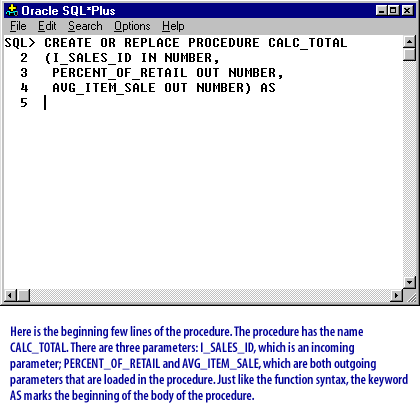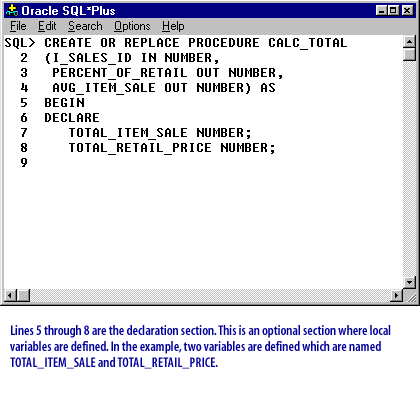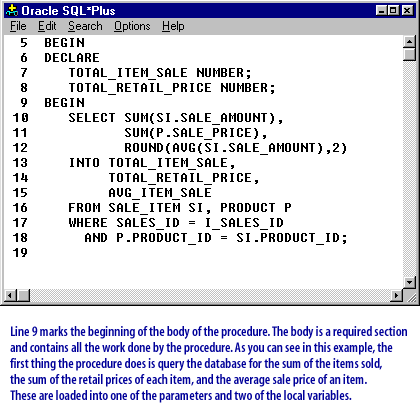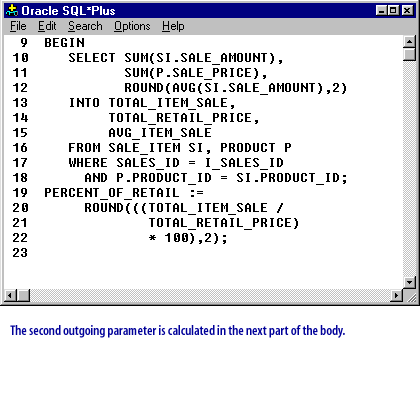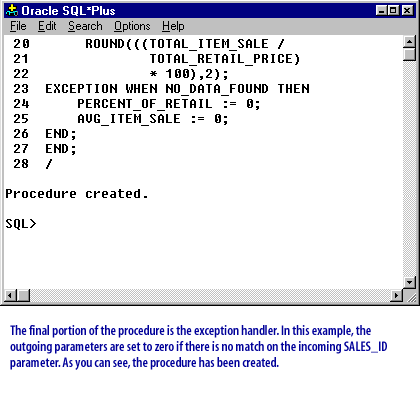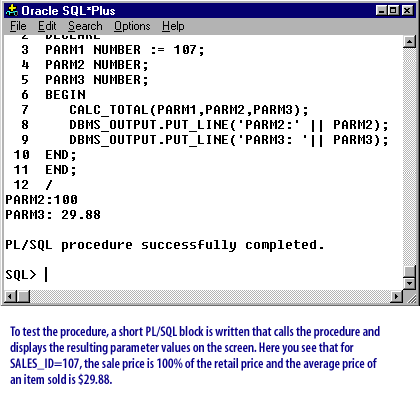Creating Oracle Procedure
The next lesson examines the capabilities and restrictions of calling other procedures.
Click the link below to create a procedure for the course project.
Create Replace Procedure

## Built-in SQL functions

Question: How do you write your own built-in SQL functions?
Answer: A function is a PL/SQL named block that returns a value. It is commonly used to convert or assign values.
Note the following: 1) A procedure is executed, 2) a function is called, as in the example below:
```Begin
get_area(11,22,n_area);
n_area := calc_area(11,22);
End;
```

In the code fragment above, the area is calculated using a procedure named `get_area` and a function named `calc_area`.
The procedure was passed three values and it copied the calculated area into the `n_area` variable when the procedure exited. The next line uses a function that is
1. passed two values,
2. calculates the area, and
3. returns that value,
which is assigned to the `n_area` variable.
Notice that the function is used directly in the assignment operation. A function is defined in the format below.

```create or replace function <Name>
(<variable list>) return <datatype>
as (or is)

local variable declaration
begin
code section
exceptions
end;
```